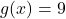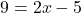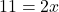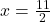## 1-25. Given f(x) =- 2/3x +3 and g(x) = 2×2 – 5, complete parts (a) through (f). Homework Help a. Calculate f(3). b. Solve

Question

1-25. Given f(x) =- 2/3x +3 and g(x) = 2×2 – 5, complete parts (a) through (f).
Homework Help
a. Calculate f(3).
b. Solve f(x)=-5.
C. Calculate g(-3).
d. Solve g(x)=-7.
e. Solve g(x)=8.
f. Solve g(x)=9.

in progress 0
1 week 2021-09-04T18:06:33+00:00 1 Answers 0 views 0

When f or g are equivalent to a number you are solving for x. For example b, d, e, f. In the equation g(x) =2×2-5 I assumed 2 was a typo. So, if it’s incorrect, please use same steps and just adjust the solution.

Step-by-step explanation: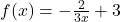a)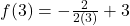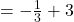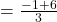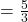b)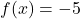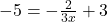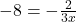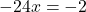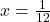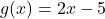c)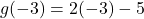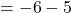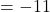d)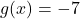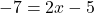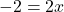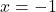e)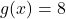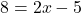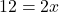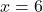f)